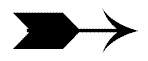Initializing Multi-Dimensional Arrays

<apmatrix.h>apmatrix variables are declared in a similar manner to apvector variables, but they work with two arguments:  the number of rows, followed by the number of columns.

//declare a matrix of doubles with 5 rows and 3 columns
apmatrix <double> table(5 , 3);You can add a third argument when declaring matrices. This "fill" value must be of the same "type" as the matrix variables.

//declare and fill matrix with astericks
apmatrix  <char> stars(2, 80, '*');Instead of the length( ) function we saw in the apvector class, the apmatrix class provides two functions for accessing the dimensions of a matrix:  numrows( ) and numcols( ).  These member functions of the apmatrix class are called using "dot" notation. (Notice the use of the nested loops.)

//function to return the sum of the elements in the matrix "table"
{
int nRows = table.numrows( );
int nCols = table.numcols( );
int row, col, sum = 0;
for(row = 0; row < nRows; row++)
{
for(col = 0; col < nCols; col++)
{
sum += table[row][col];
}
}
return sum;
}As with one-dimensional arrays, matrices can be filled one element at a time after declaration, by assignment or by user input.  Here is an example with user input.  (Notice the nested loops.)

apmatrix<int> ClubScores(4 , 5);      // declaring the 2-D array
int rows, columns;
for (rows = 0; rows < 4; rows++)     // loop for establishing the rows
{
for (columns =0; columns < 5; columns++)     // loop for the columns
{
cout << "Please enter a score ===> ";
cin >> ClubScores[rows] [columns];
}
}Printing Multi-dimensional Arrays  Nested loops are also used to print a matrix:

apmatrix<int> ClubScores(4 , 5);
int rows, columns;
for (rows = 0; rows < 4; rows++)
{
for (columns =0; columns < 5; columns++)
{
cout << ClubScores[rows] [columns] << " "; // put in spaces between..
}                                                                                    // columns when printing
cout << endl;       // a new line is needed to go to the next row
}